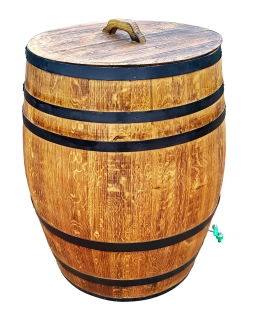# One-third of a drum

1/3 rd of a drum is filled with water . Needed another 60 l water to filled whole drum, then what is capacity of drum?

V =  90 l

### Step-by-step explanation:Did you find an error or inaccuracy? Feel free to write us. Thank you!

Tips for related online calculators
Need help calculating sum, simplifying, or multiplying fractions? Try our fraction calculator.
Do you have a linear equation or system of equations and looking for its solution? Or do you have a quadratic equation?